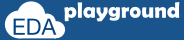# Bidirectional Constraints

SystemVerilog constraints are solved bidirectionally, which means constraints on all random variables will be solved parallel.

Consider a constraint example,

`constraint c_name { if(a == 0) b == 1;`
```                    else       b == 0; }
```

We see that 'b' is dependent on 'a'.
but constraint solver see's it as 'a' is dependent on 'b' and 'b' is dependent on 'a'.
i.e if 'b' is inline constrained as '1', in order to satisfy, 'a' should take the value '0'.

As constraints are considered from all the aspects, SystemVerilog constraints are called as bidirectional constraints.

## Bidirectional constraint example

In the example below,

Value of a should be sum of b and c, b should be greater than 6 and c should be less than 8. so in this case constraint solver will choose a value to 'a' in such a way that it should be sum of b and c, also 'b' and 'c' should satisfies their constraint.

```class packet;
rand bit [3:0] a;
rand bit [3:0] b;
rand bit [3:0] c;

constraint a_value { a == b + c; }
constraint b_value { b > 6; }
constraint c_value { c < 8; }
endclass

module bidirectional_constr;
initial begin
packet pkt;
pkt = new();
```
```    repeat(5) begin
pkt.randomize();
\$display("Value of a = %0d \tb = %0d \tc =%0d",pkt.a,pkt.b,pkt.c);
end
end
endmodule```

Simulator Output

Value of a = 8 b = 8 c =0
Value of a = 0 b = 14 c =2
Value of a = 14 b = 14 c =0
Value of a = 6 b = 15 c =7
Value of a = 13 b = 11 c =2
Execute the above code onExample-2:

In below example,

Generation of value for b is depending on value of a.
i.e if(a == 0)  b = 1;

this condition can be re-written as,
if(b == 1)  a = 0;

but there is a constraint for 'a' that value for 'a' should be always '1'. so 'b' should not take value of '1' (to satisfy constraint if(a == 0) b == 1;)

What if we make 'b' value as '1' with inline constraint?
See the below example for the answer.

```class packet;
rand bit a;
rand bit b;

constraint a_value { a == 1; }
constraint b_value { if(a == 0) b == 1;```
```                       else       b == 0; }
endclass

module bidirectional_const;
initial begin
packet pkt;
pkt = new();
```
```    pkt.randomize() with { b == 1; };
\$display("Value of a = %0d \tb = %0d",pkt.a,pkt.b);
end
endmodule```

Simulator Output

Error-[CNST-CIF] Constraints inconsistency failure
testbench.sv, 18
Constraints are inconsistent and cannot be solved.
Please check the inconsistent constraints being printed above and rewrite
them.
Execute the above code on